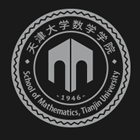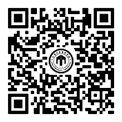﻿On some problems on the distribution of digits of integers-天津大学数学学院

# On some problems on the distribution of digits of integers

2019-10-09 15:14

For $\leq$, let $S_q (n)$ denote the sum of digits of an integer $n$ in the base $q$ expansion. Answering, in an extended form, a question of Deshouillers, Habsieger, Laishram, and Landreau, we show that, provided $a$ and $b$ are multiplicatively independent, any positive real number is a limit point of the sequence $\{ S_b (n) / S_a (n)\} _ {n \leq 1}$ . We also provide bounds for the counting functions of the corresponding subsequences. The proof uses exponential sums, discrepancy estimates and transcendence theory. This is joint work with R. de la Bretèche and G. Tenenbaum.### 联系我们